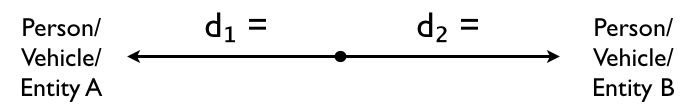Learn the 4 Things You Must Know Before Taking the GMAT!   Download it Now!

# Motion Problems: Opposite Directions

Motion problems are among the most common word problems you’ll encounter on the Quantitative section of the GMAT. These problem solving questions fall into one of three categories:

1. Motion in Opposite Directions
2. Motion in the Same Direction (“Catch Up” Problems)
3. Round Trip Questions

In this lesson we’ll cover Motion in Opposite Directions.

### 1. Motion in Opposite Directions: The Basics

All types of motion questions on the GMAT require you to apply the following basic Distance Formula:

Distance = Rate * Time

The general idea with Opposite Direction motion questions is that you have two entities…get this…moving in opposite directions from each other! One entity will be moving at a certain rate of speed, while the other entity will generally be moving at a different rate of speed (faster or slower). They may or may not start traveling at the same time. They may or may not travel the same distance.

Regardless of what information is given to you and what you’re ultimately asked to solve for, these questions always come down to solving for one of the variables in the Distance Formula. There are three crucial steps to finding the missing piece that the question is asking you to solve for.

### The Strategy

Step 1: Draw a diagram illustrating what the problem is telling you. This will help you visualize what you’re solving for and get your mind around the question itself.

The diagram will usually look something like this:Note: Sometimes the question will explain that the two entities are actually starting far away from each other and traveling towards each other. You might think of this as a “Convergence” question, but it works the exact same way as an Opposite Directions question. When you draw your diagram, just make sure the arrows point toward each other instead of away from each other as in the figure above.

Step 2: The best starting point for solving Opposite Direction questions is to use this formula:

d + d₂ = Total Distance

Step 3: Set up an individual distance formula for each entity, d₁ and d₂, and set their sum equal to the total distance traveled. You may actually be asked to find that total distance, or else the total distance will be given and you’ll have to find one of the other variables in that equation.

### On the GMAT

This will all make more sense once you see how this strategy is applied to a sample GMAT question. Watch this video for a step-by-step application: